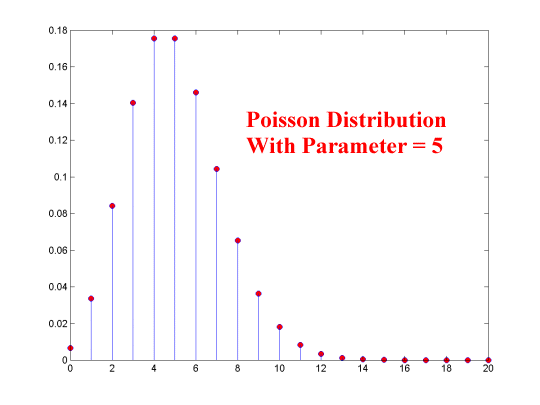LEGACY CONTENT. If you are looking for Voteview.com, PLEASE CLICK HERE

This site is an archived version of Voteview.com archived from University of Georgia on May 23, 2017. This point-in-time capture includes all files publicly linked on Voteview.com at that time. We provide access to this content as a service to ensure that past users of Voteview.com have access to historical files. This content will remain online until at least January 1st, 2018. UCLA provides no warranty or guarantee of access to these files.

### 45-733 PROBABILITY AND STATISTICS I

The Poisson Distribution

```
æ [(lx)e-l]/x!
ç
f(x) = ç            x=0,1,2,3,4,...
ç
è 0 otherwise
```

Mean = E(X) = l; and Variance = VAR(X) = l

where Lambda, l, is the average number of occurrences of the phenomenon in 1 unit of time, space, or volume.The classic example of the Poisson distribution is the distribution of deaths in the Prussian Calvary due to horse-kicks to the head. The table below shows deaths from horse-kicks 10 Army Corps over a 20 year period for a total of 200 "Corps-Years".
```
Number Reports
With This
Deaths  Many Deaths    Proportion
---------------------------------
0        109          .545
1         65          .325
2         22          .110
3          3          .015
4          1          .005
---------------------------------
200         1.000
```
It is reasonable to assume that deaths from horse-kicks should occur randomly over time. Consequently, the number per Corps-Year should have a Poisson distribution. To test this, we can treat the above data as a random sample and compute the sample mean. Using the sample mean to estimate l, we can compute the Poisson probabilities from this l and compare them to the actual frequencies in the above table and see how closely they match.

_
Xn = [åi=1,n Xi]/n = (total deaths)/(200 Corps-Years) =
(0*109 + 1*65 + 2*22 + 3*3 + 4*1)/200 = 122/200 = .61

Using l = .61 produces the following probabilities:
```
Number Reports
With This
Deaths  Many Deaths    Proportion  l=.61
----------------------------------------
0        109          .545        .543
1         65          .325        .331
2         22          .110        .101
3          3          .015        .021
4          1          .005        .003
----------------------------------------
200         1.000        .999
```
Note that only .001 of the Poisson probability is above X=4.

#### The Poisson Distribution and its Relationship to the Exponential Distribution

The Poisson Distribution can also be interpreted in the following form:
```
æ [(lt)xe-lt]/x!
ç
f(x) = ç            x=0,1,2,3,4,...
ç
è 0 otherwise
```

Mean = E(X) = lt; and Variance = VAR(X) = lt2

where l is, as above, the average number of occurrences in one unit of time, and t is the length of the time period.

Let T denote the amount of time until the first occurrence. If T > t then there must have been no occurrences in the first t units of time. The probability of this event is:

P(T > t) = P(X = 0)= e-lt

and by the rule of the complement:

P(T £ t) = 1 - e-lt

The distribution function for T is therefore:

```
æ  0  t < 0
F(t) = ç
è 1 - e-lt  t ³ 0
```
Since this is a continuous function, we can take the derivative and obtain the distribution:
```
æ  le-lt  t ³ 0
f(t) = ç
è  0  otherwise
```
Where Mean = E(T) = 1/l; and Variance = VAR(T) = 1/l2

This is the Exponential Distribution. It is the distribution of the time until the first occurrence of an event or the distribution of the amount of time between events where the occurrences of the phenomenon are governed by a Poisson process.

For example, suppose the arrival of cars at a Turnpike entrance is governed as a Poisson process with a mean of 3 per minute. Suppose the ticket taker has to leave the booth for 1 minute. What is the probability that no cars arrive during the ticket taker's absence?

P(T > 1) = 1 - F(1) = e-3*1 = .04979

As shown by this simple example the Exponential and Poisson distributions have many useful applications to what are known as Waiting Time Problems. For example, the arrival of ships at a port (both number and the time in between the arrivals); the arrival of freight trains at a classification yard; etc. The examples are legion.